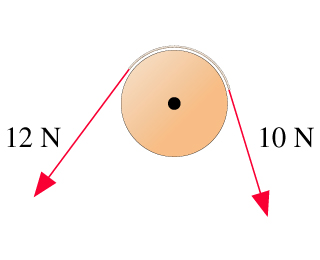# Problem: A frictionless pulley, which can be modeled as a 0.90 kg solid cylinder with a 0.31 m radius, has a rope going over it, as shown in the figure. The tensions in the rope are 12N and 10N. What is the angular acceleration of the pulley?

###### FREE Expert Solution

Torque, τ:

$\overline{){\mathbit{\tau }}{\mathbf{=}}{\mathbit{\alpha }}{\mathbit{I}}}$

or

$\overline{){\mathbit{\tau }}{\mathbf{=}}{\mathbit{r}}{\mathbit{F}}}$

The tensions are in the opposite angular direction.

ΣF = T2 - T1 = 12 - 10 = 2N

τ = (0.31)(2) = 0.62 N•m###### Problem Details

A frictionless pulley, which can be modeled as a 0.90 kg solid cylinder with a 0.31 m radius, has a rope going over it, as shown in the figure. The tensions in the rope are 12N and 10N. What is the angular acceleration of the pulley?